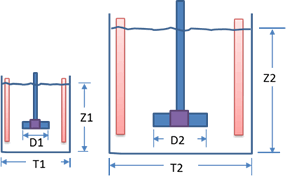# Agitation Scaleup Calculation

This web application does the scaleup from smaller to larger scale based on criteria like equal tip speed, power per unit volume, equal Reynold's number, equal shaft speed.

Reactor Geometrymm
mm
rpm

Fluid Properties

kg/m³
cP
times

Result

Shaft speed scaling is done based on following relation:

The value of n, called the scaling exponent, depends on the physical result to be scaled.

Units Base Case Scale up
Volume V m3 400 300
Vessel Diameter T mm 400 300
Liquid Level Z mm 400 300
Agitator Diameter D mm 400 300
Equivalent Diameter Teq = (4.V/π)1/3 mm 400 300
Scaling Exponent n 2
Shaft Speed N rpm 400 300
Reynold's No. Re = D2.N.ρ/μ
Tip Speed π.D.N m/s
Flow Number Nq
Pumping Rate Q = Nq.N.D3 m3/s 400 300
Power Number Np
Power Drawn P = Np.ρ.N3.D5 kW 400 300
Power/ Volume P/V kW / m3 400 300
Froude Number Fr = N2.D/g
Bulk Velocity Vc = 4.Q/(π.Teq2) m/s 400 300
Scale of Agitation 400 300